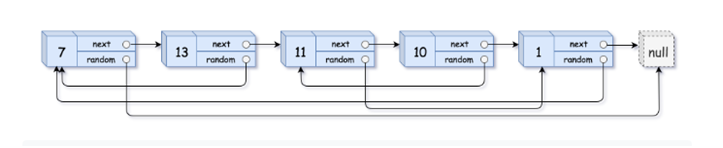## Java Memory Management

Java uses an automatic memory management system called a garbage collector

## Kahn’s Algorithm for Topological Sort

Implementation:

BFS + Queue

We will have a Adjacency List, on array which consists of degree of each vertex and a Queue

Let’s see for traverse this algorithm for the graph given below.

## Topological Sort

Topological sorting for any graph is possible if it is a DAG

Directed Acyclic Graph (DAG)

A directed graph with no cycle is called DAG

Topological Sort is the linear ordering of the vertices such that for every directed edge UV(U->V) vertex U comes before V in the ordering.

## Concurrency

Concurrency means allowing more than one transaction to operate simultaneously on a same database.

1. Reduces waiting time

2. Reduces the Response time.

3. Increases resource utilization

4. Increases Efficiency.

1.

What is Dart?

## Clone a linked list with next and random pointer with O(1) space complexity.

### We have a double linked list where each node consists of three pointers data, random and next. Now our task is to clone this linked list

Now let’s look into another solution of this problem.

## Clone a linked list with next and random pointer

### We have a double linked list where each node consists of three pointers data, random and next. Now our task is to clone this linked listLet’s first dive deep into the 1st solution of this problem

This method stores the next and random pointer (of original list) in an array first, then modifies the original Linked List (to create copy), creates a copy. And finally restores the original list.

## Backtracking AlgorithmsBacktracking is a form of recursion. But it involves choosing only option out of any possibilities. We begin by choosing an option and backtrack from it, if we reach a state where we conclude that this specific option does not give the required solution. We repeat these steps by going across each available option until we get the desired solution.

This technique use brute force approach which says that for a given problem you should try all possible solutions and pick up desired solutions

## Java Streams### Overview

The addition of the Stream was one of the major features added to Java 8. This in-depth tutorial is an introduction to the many functionalities supported by streams, with a focus on simple, practical examples.

### Introduction

First of all, Java 8 Streams should not be confused with Java I/O streams (ex: FileInputStream etc); these have very little to do with each other.

Simply put, streams are wrappers around a data source, allowing us to operate with that data source and making bulk processing convenient and fast.

# Fundamentals - I

## Motivations for Concurrency

There are a number of motivations for the usage of Concurrency in today's time, and in many cases now more than ever we are living in a time where concurrency has become one of the most important factor in the scaling factors of most of the applications that we develop.

Subscribe to Code Standards